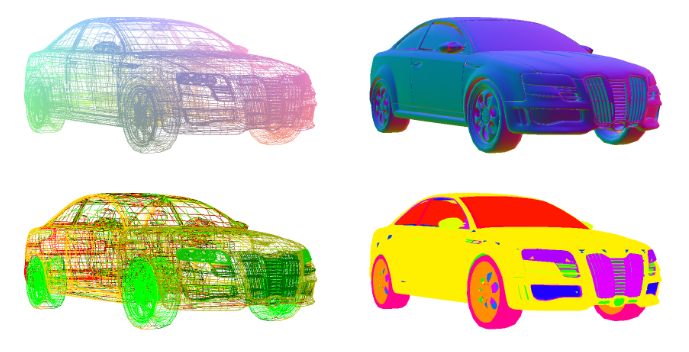### 3D.Compute.Render.MeshOrbit

This node renders a 3D mesh with an orbiting camera. It is intended for debugging the information contained in a mesh node (such as positions, per-vertex normals, and texture coordinates).camera
mesh bounding box
$d$ largest box dimension
orbit path
$r=1.75\,d$
$x$
$y$
$z$

As shown in the figure above, the virtual camera is rotating around the 3D mesh on a circular orbiting path with a radius of $r=1.75\,d$, where $d$ is the largest dimension of the axis-aligned bounding box for the 3D mesh. The camera has a fixed vertical field of view angle of 60 degrees.

The orbit plane input slot selects the plane in which the orbit path lies ($x$-$y$, $x$-$z$, or $y$-$z$). In the figure above this is the $x$-$y$-plane.

The orbit offset input slot offsets the orbit path in a perpendicular direction to the selected orbit plane.

The type input slot selects which attribute of the mesh is shown color-coded as RGB:

• Type = 0: Position ($x$, $y$, $z$ coordinates of the vertices within the mesh's axis-aligned bounding box)
• Type = 1: Normal (per-vertex normals)
• Type = 2: TexCoord ($s$, $t$ texture coordinates)
• Type = 3: Group (mesh group index)

The wireframe input slot allows rendering the mesh in wireframe mode, which is useful for debugging the mesh's connectivity information.The image above shows color-coded positions (top left), color-coded normals (top right), color-coded texture coordinates (bottom left) and color coded mesh groups (bottom right). For postions and texture coordinates the wireframe mode was enabled.